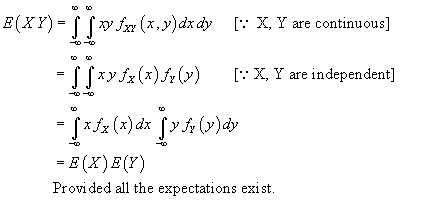Ask question

# Give a counter example to the statement E(XY)=E(X)E(Y) impliesthat X and Y are independent.# Give a counter example to the statement E(XY)=E(X)E(Y) impliesthat X and Y are independent.

Question
Random variablesasked 2021-03-07

Give a counter example to the statement $$E(XY)=E(X)E(Y)$$ implies
that X and Y are independent.

## Answers (1)2021-03-08

Suppose X and Y independent random variables, then
$$E(XY) = E(X)E(Y)$$### Relevant Questionsasked 2021-02-08

A population of values has a normal distribution with $$\displaystyle\mu={204.3}$$ and $$\displaystyle\sigma={43}$$. You intend to draw a random sample of size $$\displaystyle{n}={111}$$.
Find the probability that a single randomly selected value is less than 191.2.
$$\displaystyle{P}{\left({X}{<}{191.2}\right)}=$$?
Find the probability that a sample of size $$\displaystyle{n}={111}$$ is randomly selected with a mean less than 191.2.
$$\displaystyle{P}{\left({M}{<}{191.2}\right)}=$$?
Write your answers as numbers accurate to 4 decimal places.asked 2021-02-05

A population of values has a normal distribution with $$\displaystyle\mu={181}$$ and $$\displaystyle\sigma={41.8}$$. You intend to draw a random sample of size $$\displaystyle{n}={144}$$.
Find the probability that a single randomly selected value is between 176.5 and 183.1.
$$\displaystyle{P}{\left({176.5}{<}{M}{<}{183.1}\right)}=$$?
Write your answers as numbers accurate to 4 decimal places.asked 2020-11-30

A population of values has a normal distribution with $$\displaystyle\mu={29.3}$$ and $$\displaystyle\sigma={65.1}$$. You intend to draw a random sample of size $$\displaystyle{n}={142}$$.
Find the probability that a sample of size n=142 is randomly selected with a mean between 27.7 and 35.3.
$$\displaystyle{P}{\left({27.7}{<}\overline{{{X}}}{<}{35.3}\right)}=$$?
Write your answers as numbers accurate to 4 decimal places.asked 2020-11-29

A population of values has a normal distribution with $$\displaystyle\mu={116.5}$$ and $$\displaystyle\sigma={63.7}$$. You intend to draw a random sample of size $$\displaystyle{n}={244}$$.
Find the probability that a sample of size $$\displaystyle{n}={244}$$ is randomly selected with a mean between 104.7 and 112.8.
$$\displaystyle{P}{\left({104.7}{<}{M}{<}{112.8}\right)}=$$?
Write your answers as numbers accurate to 4 decimal places.asked 2020-12-06

A population of values has a normal distribution with $$\displaystyle\mu={13.2}$$ and $$\displaystyle\sigma={5}$$. You intend to draw a random sample of size $$\displaystyle{n}={60}$$.
Find the probability that a sample of size $$\displaystyle{n}={60}$$ is randomly selected with a mean between 11.5 and 14.
$$\displaystyle{P}{\left({11.5}{<}{M}{<}{14}\right)}=$$?
Write your answers as numbers accurate to 4 decimal places.asked 2021-02-02

A population of values has a normal distribution with $$\displaystyle\mu={13.2}$$ and $$\displaystyle\sigma={5}$$. You intend to draw a random sample of size $$\displaystyle{n}={60}$$.
Find the probability that a single randomly selected value is between 11.5 and 14.
$$\displaystyle{P}{\left({11.5}{<}{X}{<}{14}\right)}=$$?
Write your answers as numbers accurate to 4 decimal places.asked 2021-02-25

A population of values has a normal distribution with $$\displaystyle\mu={192.6}$$ and $$\displaystyle\sigma={34.4}$$. You intend to draw a random sample of size $$\displaystyle{n}={173}$$.
Find the probability that a sample of size $$\displaystyle{n}={173}$$ is randomly selected with a mean less than 186.1.
$$\displaystyle{P}{\left({M}{<}{186.1}\right)}=$$?
Write your answers as numbers accurate to 4 decimal places.asked 2020-12-06

A population of values has a normal distribution with $$\displaystyle\mu={13.7}$$ and $$\displaystyle\sigma={22}$$.
You intend to draw a random sample of size $$\displaystyle{n}={78}$$.
Find the probability that a single randomly selected value is less than 11.5.
$$\displaystyle{P}{\left({X}{<}{11.5}\right)}=$$?
Write your answers as numbers accurate to 4 decimal places.asked 2020-12-07

A population of values has a normal distribution with $$\displaystyle\mu={99.6}$$ and $$\displaystyle\sigma={35.1}$$. You intend to draw a random sample of size $$\displaystyle{n}={84}$$.
Find the probability that a single randomly selected value is between 98.5 and 100.7.
$$\displaystyle{P}{\left({98.5}{<}{X}{<}{100.7}\right)}=$$?
Write your answers as numbers accurate to 4 decimal places.asked 2021-02-05

A population of values has a normal distribution with $$\displaystyle\mu={181}$$ and $$\displaystyle\sigma={41.8}$$. You intend to draw a random sample of size $$\displaystyle{n}={144}$$.
Find the probability that a single randomly selected value is between 176.5 and 183.1.
$$\displaystyle{P}{\left({176.5}{<}{X}{<}{183.1}\right)}=$$?
Write your answers as numbers accurate to 4 decimal places.

...